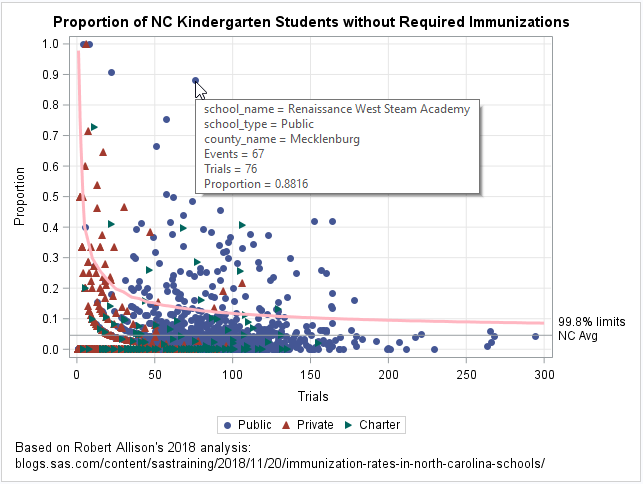Last week my colleague, Robert Allison, visualized data regarding immunization rates for kindergarten classes in North Carolina. One of his graphs was a scatter plot that displayed the proportion of unimmunized students versus the size of the class for 1,885 kindergarten classes in NC. This scatter plot is the basis for a statistical plot that is known as a funnel plot for proportions. The plot to the right shows a funnel plot that I created based on Robert's analysis.

The basic idea of a funnel plot is that small random samples have more variation than large random samples from the same population. If students are randomly chosen from a population in which some small proportion of children have an attribute, it might not be unusual if 40% of the students in a five-student class have the attribute (that's 2 out of 5) whereas it might be highly unusual to see such a high proportion in a 100-student class. The funnel plot enhances a scatter plot by adding curves that indicate a reasonable range of values for the proportion, given the size of a random sample.

For a discussion of funnel plots and how to create a funnel plot in SAS, see the article "Funnel plots for proportions." You can download the immunization data and the SAS program that I used to create the funnel plot for proportions.

A funnel plot for proportions

The preceding funnel plot contains the following statistical features:
• A scatter plot of the data. Each marker represents a school.
• A horizontal reference line. The line indicates the average proportion for the data. For these data, the statewide average proportion of unimmunized kindergarteners is 4.58%.
• A curve that indicates an upper confidence limit for the proportion of unimmunized students, assuming that the classes are a random sample from a population in which 4.58% of the students are unimmunized. When a marker (school) appears above the curve, the proportion of unimmunized students in that school is significantly higher than the statewide proportion.

This funnel plot uses the shape (and color) of a marker to indicate whether the school is public (circle), private (upward-pointing triangle), or a charter school (right-pointing triangle). The plot includes tool tips so that you can hover the mouse over a marker and see the name and county of the school.

The graph indicates that there are dozens of schools for which the proportion of unimmunized students far exceeds the state average. A graph like this enables school administrators and public health officials to identify schools that have a larger-than-expected proportion of unimmunized students. Identifying schools is the first step to developing initiatives that can improve the immunization rate in school-age children.

Funnel plots for each school district

You can use a WHERE statement or BY-group processing in PROC SGPLOT to create a funnel plot for each county. A graph that shows only the schools in a particular district is more relevant for local school boards and administrators. The following graphs show the proportion of unimmunized students in Mecklenburg County (near Charlotte) and Wake County (near Raleigh), which are the two largest school districts in NC.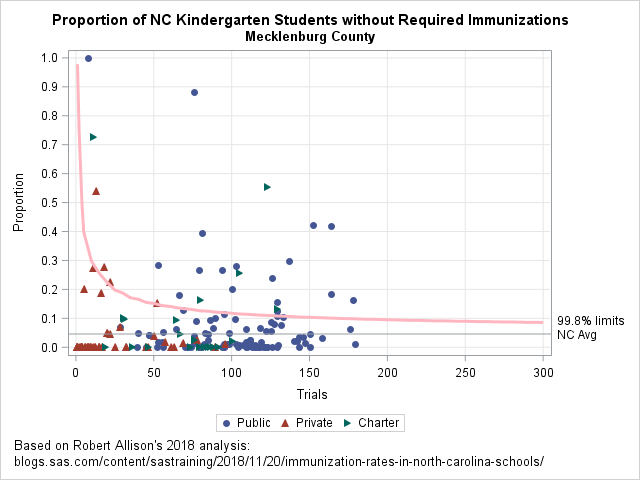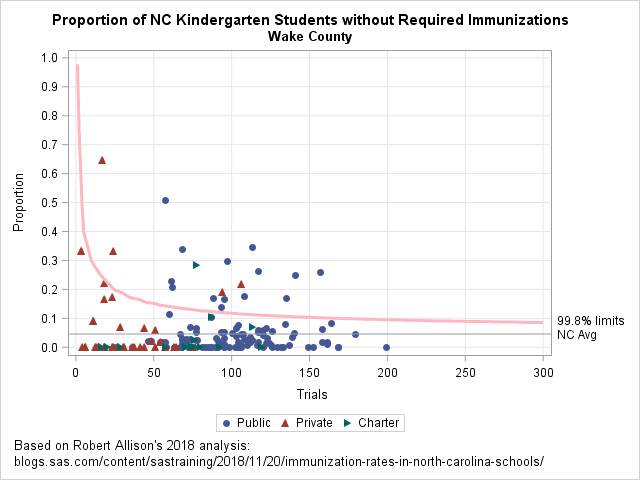The first graph shows that Mecklenburg County has several schools that contain more than 60 kindergarten students and for which 25% or more of the students are unimmunized. In fact, some large schools have more than 40% unimmunized! In Wake County, fewer schools have extreme proportions, but there are still many schools for which the proportion of unimmunized students is quite large relative to the statewide average.

As Robert pointed out in his blog post, these are not official figures, so it is possible that some of the extreme proportions are caused by data entry errors rather than by hordes of unimmunized students.

Funnel plots for public, private, and charter schools

The following graph shows a panel of plots for public, private, and charter schools. There are many public schools whose proportion of unimmunized students is well above the statewide average. For the private and charter schools, about 10 schools stand out in each group.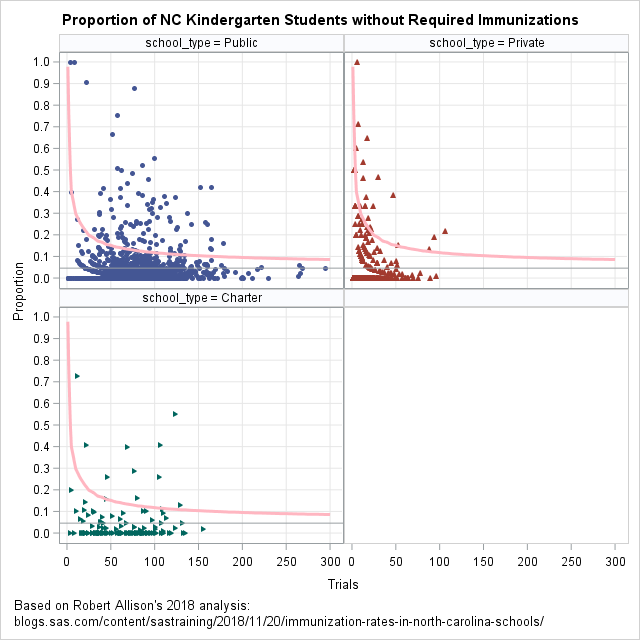I think the plot of private schools is particularly interesting. When the media reports on outbreaks of childhood diseases in schools, there is often a mention of a "religious exemption," which is when a parent or guardian states that a child will not be immunized because of their religious beliefs. The report often mentions that private schools are often affiliated with a particular religion or church. I've therefore assumed that private schools have a larger proportion of unimmunized students because of the religious exemption. These data do not indicate which students are unimmunized because of a religious exemption, but the panel of funnel plots indicates that, overall, not many private schools have an abnormally large proportion of unimmunized students. In fact, the private schools show smaller deviations from the expected value than the public and charter schools.

Summary

In summary, I started with one of Robert Allison's graphs and augmented it to create a funnel plot for proportions. A funnel plot shows the average proportion and confidence limits for proportions (given the sample size). If the students in the schools were randomly sampled from a population where 4.58% of students are unimmunized, then few schools would be outside of the confidence curve. Of course, in reality, schools are not random samples. Many features of school performance—including unimmunized students—depend on local socioeconomic conditions. By taking into account the size of the classes, the funnel plot identifies schools that have an exceptionally large proportion of unimmunized students. A funnel plot can help school administrators and public health officials identify schools that might benefit from intervention programs and additional resources, or for which the data were incorrectly entered.

The post A funnel plot for immunization rates appeared first on The DO Loop.A data analyst asked how to compute parameter estimates in a linear regression model when the underlying data matrix is rank deficient. This situation can occur if one of the variables in the regression is a linear combination of other variables. It also occurs when you use the GLM parameterization of a classification variable. In the GLM parameterization, the columns of the design matrix are linearly dependent. As a result, the matrix of crossproducts (the X`X matrix) is singular. In either case, you can understand the computation of the parameter estimates learning about generalized inverses in linear systems. This article presents an overview of generalized inverses. A subsequent article will specifically apply generalized inverses to the problem of estimating parameters for regression problems with collinearities.

What is a generalized inverse?

Recall that the inverse matrix of a square matrix A is a matrix G such as A*G = G*A = I, where I is the identity matrix. When such a matrix exists, it is unique and A is said to be nonsingular (or invertible). If there are linear dependencies in the columns of A, then an inverse does not exist. However, you can define a series of weaker conditions that are known as the Penrose conditions:

1. A*G*A = A
2. G*A*G = G
3. (A*G)` = A*G
4. (G*A)` = G*A

Any matrix, G, that satisfies the first condition is called a generalized inverse (or sometimes a "G1" inverse) for A. A matrix that satisfies the first and second condition is called a "G2" inverse for A. The G2 inverse is used in statistics to compute parameter estimates for regression problems (see Goodnight (1979), p. 155). A matrix that satisfies all four conditions is called the Moore-Penrose inverse or the pseudoinverse. When A is square but singular, there are infinitely many matrices that satisfy the first two conditions, but the Moore-Penrose inverse is unique.

Computations with generalized inverses

In regression problems, the parameter estimates are obtained by solving the "normal equations." The normal equations are the linear system (X`*X)*b = (X`*Y), where X is the design matrix, Y is the vector of observed responses, and b is the parameter estimates to be solved. The matrix A = X`*X is symmetric. If the columns of the design matrix are linearly dependent, then A is singular. The following SAS/IML program defines a symmetric singular matrix A and a right-hand-side vector c, which you can think of as X`*Y in the regression context. The call to the DET function computes the determinant of the matrix. A zero determinant indicates that A is singular and that there are infinitely many vectors b that solve the linear system:

```proc iml; A = {100 50 20 10, 50 106 46 23, 20 46 56 28, 10 23 28 14 }; c = {130, 776, 486, 243};   det = det(A); /* demonstrate that A is singular */ print det;```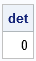For nonsingular matrices, you can use either the INV or the SOLVE functions in SAS/IML to solve for the unique solution of the linear system. However, both functions give errors when called with a singular matrix. SAS/IML provides several ways to compute a generalized inverse, including the SWEEP function and the GINV function. The SWEEP function is an efficient way to use Gaussian elimination to solve the symmetric linear systems that arise in regression. The GINV function is a function that computes the Moore-Penrose pseudoinverse. The following SAS/IML statements compute two different solutions for the singular system A*b = c:

```b1 = ginv(A)*c; /* solution even if A is not full rank */ b2 = sweep(A)*c; print b1 b2;```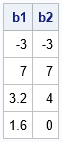The SAS/IML language also provides a way to obtain any of the other infinitely many solutions to the singular system A*b = c. Because A is a rank-1 matrix, it has a one-dimensional kernel (null space). The HOMOGEN function in SAS/IML computes a basis for the null space. That is, it computes a vector that is mapped to the zero vector by A. The following statements compute the unit basis vector for the kernel. The output shows that the vector is mapped to the zero vector:

```xNull = homogen(A); /* basis for nullspace of A */ print xNull (A*xNull)[L="A*xNull"];```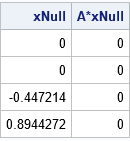All solutions to A*b = c are of the form b + α*xNull, where b is any particular solution.

Properties of the Moore-Penrose solution

You can verify that the Moore-Penrose matrix GINV(A) satisfies the four Penrose conditions, whereas the G2 inverse (SWEEP(A)) only satisfies the first two conditions. I mentioned that the singular system has infinitely many solutions, but the Moore-Penrose solution (b1) is unique. It turns out that the Moore-Penrose solution is the solution that has the smallest Euclidean norm. Here is a computation of the norm for three solutions to the system A*b = c:

```/* GINV gives the estimate that has the smallest L2 norm: */ GINVnorm = norm(b1); sweepNorm = norm(b2); /* you can add alpha*xNull to any solution to get another solution */ b3 = b1 + 2*xNull; /* here's another solution (alpha=2) */ otherNorm = norm(b3); print ginvNorm sweepNorm otherNorm;```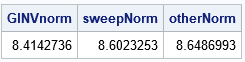Because all solutions are of the form b1 + α*xNull, where xNull is the basis for the nullspace of A, you can graph the norm of the solutions as a function of α. The graph is shown below and indicates that the Moore-Penrose solution is the minimal-norm solution:

```alpha = do(-2.5, 2.5, 0.05); norm = j(1, ncol(alpha), .); do i = 1 to ncol(alpha); norm[i] = norm(b1 + alpha[i] * xNull); end; title "Euclidean Norm of Solutions b + alpha*xNull"; title2 "b = Solution from Moore-Penrose Inverse"; title3 "xNull = Basis for Nullspace of A"; call series(alpha, norm) other="refline 0 / axis=x label='b=GINV';refline 1.78885 / axis=x label='SWEEP';";```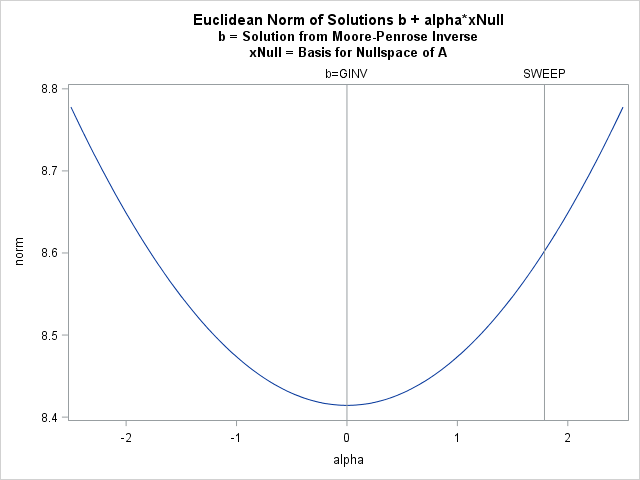In summary, a singular linear system has infinitely many solutions. You can obtain a particular solution by using the sweep operator or by finding the Moore-Penrose solution. You can use the HOMOGEN function to obtain the full family of solutions. The Moore-Penrose solution is expensive to compute but has an interesting property: it is the solution that has the smallest Euclidean norm. The sweep solution is more efficient to compute and is used in SAS regression procedures such as PROC GLM to estimate parameters in models that include classification variables and use a GLM parameterization. The next blog post explores regression estimates in more detail.

The post Generalized inverses for matrices appeared first on The DO Loop.An ROC curve graphically summarizes the tradeoff between true positives and true negatives for a rule or model that predicts a binary response variable. An ROC curve is a parametric curve that is constructed by varying the cutpoint value at which estimated probabilities are considered to predict the binary event. Most SAS data analysts know that you can fit a logistic model in PROC LOGISTIC and create an ROC curve for that model, but did you know that PROC LOGISTIC enables you to create and compare ROC curves for ANY vector of predicted probabilities regardless of where the predictions came from? This article shows how!

If you want to review the basic constructions of an ROC curve, you can see a previous article that constructs an empirical ROC curve from first principles. The PROC LOGISTIC documentation provides formulas used for constructing an ROC curve.

Produce an ROC plot by using PROC LOGISTIC

Before discussing how to create an ROC plot from an arbitrary vector of predicted probabilities, let's review how to create an ROC curve from a model that is fit by using PROC LOGISTIC. The following data and model are taken from the the PROC LOGISTIC documentation. The data are for 43 cancer patients who also had an intestinal obstruction. The response variable popInd is a postoperative indicator variable: popInd = 1 for patients who died within two months after surgery. The explanatory variables are three pre-operative screening tests. The goal of the study is to determine patients who might benefit from surgery, where "benefit" is measured by postoperative survival of at least two months.

```data roc; input alb tp totscore popind @@; totscore = 10 - totscore; datalines; 3.0 5.8 10 0 3.2 6.3 5 1 3.9 6.8 3 1 2.8 4.8 6 0 3.2 5.8 3 1 0.9 4.0 5 0 2.5 5.7 8 0 1.6 5.6 5 1 3.8 5.7 5 1 3.7 6.7 6 1 3.2 5.4 4 1 3.8 6.6 6 1 4.1 6.6 5 1 3.6 5.7 5 1 4.3 7.0 4 1 3.6 6.7 4 0 2.3 4.4 6 1 4.2 7.6 4 0 4.0 6.6 6 0 3.5 5.8 6 1 3.8 6.8 7 1 3.0 4.7 8 0 4.5 7.4 5 1 3.7 7.4 5 1 3.1 6.6 6 1 4.1 8.2 6 1 4.3 7.0 5 1 4.3 6.5 4 1 3.2 5.1 5 1 2.6 4.7 6 1 3.3 6.8 6 0 1.7 4.0 7 0 3.7 6.1 5 1 3.3 6.3 7 1 4.2 7.7 6 1 3.5 6.2 5 1 2.9 5.7 9 0 2.1 4.8 7 1 2.8 6.2 8 0 4.0 7.0 7 1 3.3 5.7 6 1 3.7 6.9 5 1 3.6 6.6 5 1 ;   ods graphics on; proc logistic data=roc plots(only)=roc; LogisticModel: model popind(event='0') = alb tp totscore; output out=LogiOut predicted=LogiPred; /* output predicted value, to be used later */ run;```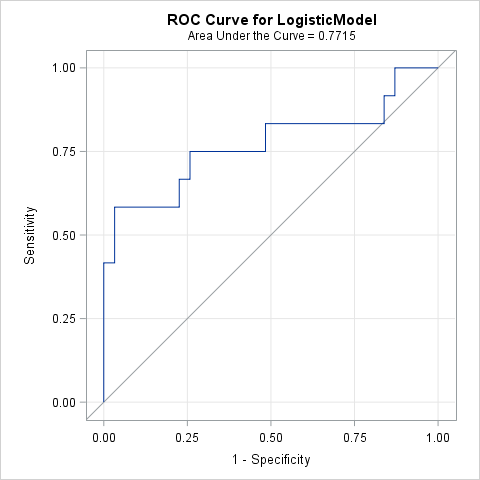You can see the documentation for details about how to interpret the output from PROC LOGISTIC, but the example shows that you can use the PLOTS=ROC option (or the ROC statement) to create an ROC curve for a model that is fit by PROC LOGISTIC. For this model, the area under the ROC curve is 0.77. Because a random "coin flip" prediction has an expected area of 0.5, this model predicts the survival of surgery patients better than random chance.

Create an ROC curve for any prediction rule

A logistic model is not the only way to predict a binary response. You could also use a decision tree, a generalized mixed model, a nonparametric regression model, or even ask a human expert for her opinion. An ROC curve only requires two quantities: for each observation, you need the observed binary response and a predicted probability. In fact, if you carefully read the PROC LOGISTIC documentation, you will find these sentences:

• In the "Details" section: "ROC curves can be created ... from the specified model in the MODEL statement, from specified models in ROC statements, or from input variables which act as [predicted probabilities]." (Emphasis added.)
• In the documentation of the ROC statement: "The PRED= option enables you to input a criterion produced outside PROC LOGISTIC; for example, you can fit a random-intercept model by using PROC GLIMMIX or use survey weights in PROC SURVEYLOGISTIC, then use the predicted values from those models to produce an ROC curve for the comparisons."

In other words, you can use PROC LOGISTIC to create an ROC curve regardless of how the predicted probabilities are obtained! For argument's sake, let's suppose that you ask a human expert to predict the probability of each patient surviving for at least two months after surgery. (Notice that there is no statistical model here, only a probability for each patient.) The following SAS DATA step defines the predicted probabilities, which are then merged with the output from the earlier PROC LOGISTIC call:

```data ExpertPred; input ExpertPred @@; datalines; 0.95 0.2 0.05 0.3 0.1 0.6 0.8 0.5 0.1 0.25 0.1 0.2 0.05 0.1 0.05 0.1 0.4 0.1 0.2 0.25 0.4 0.7 0.1 0.1 0.3 0.2 0.1 0.05 0.1 0.4 0.4 0.7 0.2 0.4 0.1 0.1 0.9 0.7 0.8 0.25 0.3 0.1 0.1 ; data Survival; merge LogiOut ExpertPred; run;   /* create ROC curve from a variable that contains predicted values */ proc logistic data=Survival; model popind(event='0') = ExpertPred / nofit; roc 'Expert Predictions' pred=ExpertPred; ods select ROCcurve; run;```Notice that you only need to supply two variables on the MODEL statements: the observed responses and the variable that contains the predicted values. On the ROC statement, I've used the PRED= option to indicate that the ExpertPred variable is not being fitted by the procedure. Although PROC LOGISTIC creates many tables, I've used the ODS SELECT statement to suppress all output except for the ROC curve.

Overlay and compare ROC curves from different models or rules

You might want to overlay and compare ROC curves from multiple predictive models (either from PROC LOGISTIC or from other sources). PROC LOGISTIC can do that as well. You just need to merge the various predicted probabilities into a single SAS data set and then specify multiple ROC statements, as follows:

```/* overlay two or more ROC curves by using variables of predicted values */ proc logistic data=Survival; model popind(event='0') = LogiPred ExpertPred / nofit; roc 'Logistic' pred=LogiPred; roc 'Expert' pred=ExpertPred; ods select ROCOverlay; /* optional: for a statistical comparison, use ROCCONTRAST stmt and remove the ODS SELECT stmt */ *roccontrast reference('Expert Model') / estimate e; run;```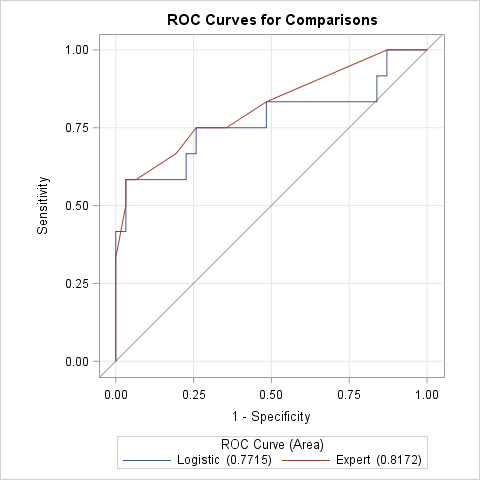This ROC overlay shows that the "expert" prediction is almost always superior or equivalent to the logistic model in terms of true and false classification rates. As noted in the comments of the previous call to PROC LOGISTIC, you can use the ROCCONTRAST statement to obtain a statistical analysis of the difference between the areas under the curves (AUC).

In summary, you can use the ROC statement in PROC LOGISTIC to generate ROC curves for models that were computed outside of PROC LOGISTIC. All you need are the predicted probabilities and observed response for each observation. You can also overlay and compare two or more ROC curves and use the ROCCONTRAST statement to analyze the difference between areas under the curves.

The post Create and compare ROC curves for any predictive model appeared first on The DO Loop.Will the real Pareto distribution please stand up?

SAS supports three different distributions that are named "Pareto." The Wikipedia page for the Pareto distribution lists five different "Pareto" distributions, including the three that SAS supports. This article shows how to fit the two-parameter Pareto distribution in SAS and discusses the relationship between the "standard" Pareto distribution and other "generalized" Pareto distributions.

The Pareto distribution

To most people, the Pareto distribution refers to a two-parameter continuous probability distribution that is used to describe the distribution of certain quantities such as wealth and other resources. This "standard" Pareto is sometimes called the "Type I" Pareto distribution.

The SAS language supports the four essential functions for working with the Type I Pareto probability distribution (PDF, CDF, quantiles, and random number generation). The Pareto density function is usually written as
f(x) = α xmα / xα + 1
where the shape parameter α > 0 describes how quickly the density decays and the scale parameter xm > 0 is the minimum possible value of x. The Roman letter a is sometimes used in place of the Greek letter α. The following graph shows three density curves for xm = 1.5 and for three values of a (or α):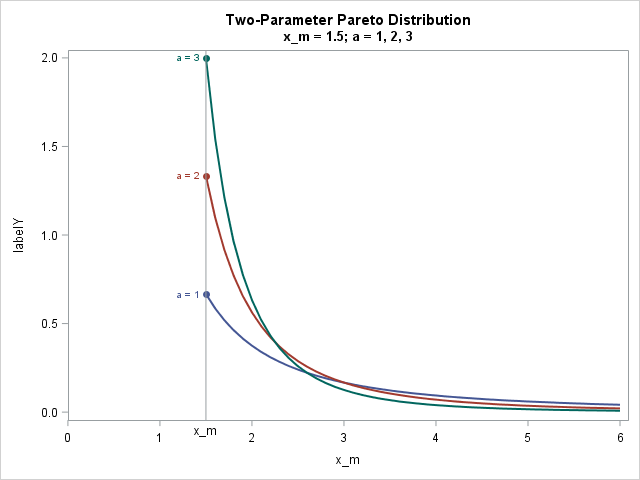Fit the Pareto distribution in SAS

There are two ways to fit the standard two-parameter Pareto distribution in SAS. It turns out that the maximum likelihood estimates (MLE) can be written explicitly in terms of the data. Therefore, you can use SAS/IML (or use PROC SQL and the DATA step) to explicitly compute the estimates, as shown below:

```/* MLE estimate for standard two-parameter Pareto. For the formula, see https://en.wikipedia.org/wiki/Pareto_distribution#Parameter_estimation */ proc iml; use Pareto; read all var "x"; close; x_m = min(x); /* MLE estimate of scale param */ a = countn(x) / sum(log(x/x_m)); /* MLE estimate of shape param */ Est = x_m || a; print Est[c={"x_m" "a"} L="Pareto MLE Estimates"]; quit;```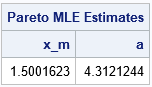The second way to fit the Pareto distribution is to use PROC NLMIXED, which can fit general MLE problems. You need to be a little careful when estimating the x_m parameter because that parameter must be less than or equal to the minimum value in the data. In the following, I've used a macro variable that contains the minimum value of the data to constrain the MLE estimates to be in the interval (0, min(x)]:

```proc sql; select Min(x) into :minX from Pareto; /* store x_m in macro */ run; proc nlmixed data=Pareto; parms x_m 1 a 2; * initial values of parameters; bounds 0 < x_m < &minX, a > 0; * bounds on parameters; LL = log(a) + a*log(x_m) - (a+1)*log(x); * or use logpdf("Pareto", x, a, x_m); model x ~ general(LL); run;```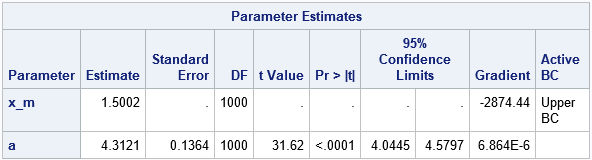Notice that the MLE estimates optimize the constrained log-likelihood (LL) function, but the gradient does not vanish at the optimal parameter values. This explains why the output from PROC NLMIXED does not include an estimate for the standard error or confidence intervals for the x_m parameter. You do, however, get an estimate for the standard error of the shape parameter, which is not available from the direct computation of the point estimate.

If you overlay a histogram and the density estimate for the fitted parameters, you obtain the following graph, which shows that the Pareto curve fits the data well.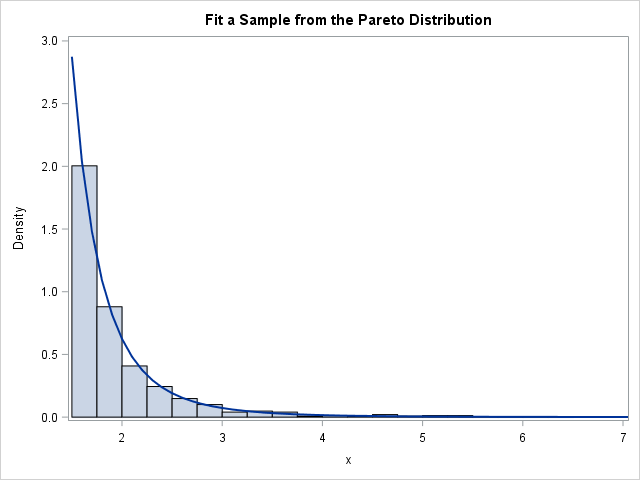Pareto versus generalized Pareto distributions

The previous section shows how to fit the two-parameter (Type I) Pareto distribution in SAS. Unfortunately, some SAS programmers see that PROC UNIVARIATE supports a PARETO option on the HISTOGRAM statement, and assume that it is the usual two-parameter Pareto distribution. It is not. The UNIVARIATE procedure supports a generalized Pareto distribution, which is different from ==the standard distribution.

The UNIVARIATE procedure fits a generalized (Type II) Pareto distribution, which has three parameters: the threshold parameter θ, the scale parameter σ, and a shape parameter. Unfortunately, the UNIVARIATE documentation refers to the shape parameter as α, but it is not the same parameter as in the standard two-parameter Pareto distribution. In particular, the shape parameter in PROC UNIVARIATE can be positive or negative. The three-parameter generalized (Type II) Pareto distribution reduces to the standard Pareto when θ = -σ / α. Call that common value xm and define β = -1 / α. Then you can show that the PDF of the generalized Pareto (as supported in PROC UNIVARIATE) reduces to the standard Type I Pareto (which is supported by the PDF, CDF, and RAND functions). In general, however, the three-parameter generalized Pareto distribution can fit a wider variety of density curves than the two-parameter Pareto can.

The UNIVARIATE parameterization is similar to the parameterization for the Type II generalized Pareto distribution that is listed in the Wikipedia article. If you start with the UNIVARIATE parameterization and define s = -σ / α and β = -1 / α, then you obtain the parameterization in the Wikipedia article.

You can use PROC NLMIXED to fit any of the generalized Pareto distributions. I've already shown how to fit the Type I distribution. Two other procedures in SAS support other generalized Pareto distributions:

It is a real annoyance to have so many different versions of the Pareto distribution, but such is life. With apologies to the Gershwin brothers, I'll end with a reference to the old song, "Let's Call the Whole Thing Off":

You say Pareto, and I say Par-ah-to.
Pareto, Par-ah-to, generalized Pareto?
Let's call the whole thing off!

If you don't want to "call the whole thing off," hopefully this article explains the different forms of the Pareto distributions in SAS and how to estimate the parameters in these models.

The post Fit the Pareto distribution in SAS appeared first on The DO Loop.In a recent article about nonlinear least squares, I wrote, "you can often fit one model and use the ESTIMATE statement to estimate the parameters in a different parameterization." This article expands on that statement. It shows how to fit a model for one set of parameters and use the ESTIMATE statement to obtain estimates for a different set of parameters.

A canonical example of a probability distribution that has multiple parameterizations is the gamma distribution. You can parameterize the gamma distribution in terms of a scale parameter or in terms of a rate parameter, where the rate parameter is the reciprocal of the scale parameter. The density functions for the gamma distribution with scale parameter β or rate parameter c = 1 / β are as follows:
Shape α, scale β: f(x; α, β) = 1 / (βα Γ(α)) xα-1 exp(-x/β)
Shape α, rate c: f(x; α, c) = cα / Γ(α) xα-1 exp(-c x)

You can use PROC NLMIXED to fit either set of parameters and use the ESTIMATE statement to obtain estimates for the other set. In general, you can do this whenever you can explicitly write down an analytical formula that expresses one set of parameters in terms of another set.

Estimating the scale or rate parameter in a gamma distribution

Let's implement this idea on some simulated data. The following SAS DATA step simulates 100 observations from a gamma distribution with shape parameter α = 2.5 and scale parameter β = 1 / 10. A call to PROC UNIVARIATE estimates the parameters from the data and overlays a gamma density on the histogram of the data:

```/* The gamma model has two different parameterizations: a rate parameter and a scale parameter. Generate gamma-distributed data and fit a model for each parameterization. Use the ESTIMATE stmt to estimate the parameter in the model that was not fit. */ %let N = 100; /* N = sample size */ data Gamma; aParm = 2.5; scaleParm = 1/10; rateParm = 1/scaleParm; do i = 1 to &N; x = rand("gamma", aParm, scaleParm); output; end; run;   proc univariate data=Gamma; var x; histogram x / gamma; run;```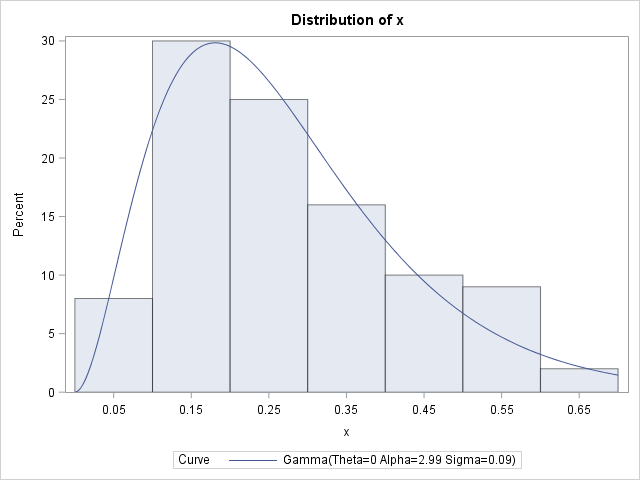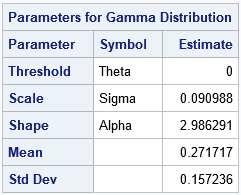The parameter estimates are (2.99, 0.09), which are close to the parameter values that were used to generate the data. Notice that PROC UNIVARIATE does not provide standard errors or confidence intervals for these point estimates. However, you can get those statistics from PROC NLMIXED. PROC NLMIXED also supports the ESTIMATE statement, which can estimate the rate parameter:

```/*Use PROC NLMIXED to fit the shape and scale parameters in a gamma model */ title "Fit Gamma Model, SCALE Parameter"; proc nlmixed data=Gamma; parms a 1 scale 1; * initial value for parameter; bounds 0 < a scale; model x ~ gamma(a, scale); rate = 1/scale; estimate 'rate' rate; ods output ParameterEstimates=PE; ods select ParameterEstimates ConvergenceStatus AdditionalEstimates; run;```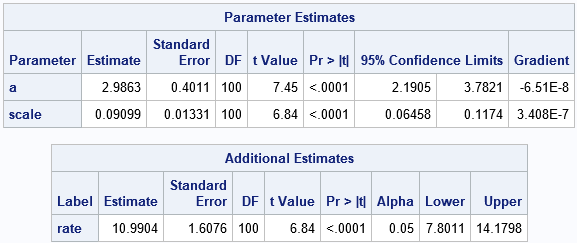The parameter estimates are the same as from PROC UNIVARIATE. However, PROC NLMIXED also provides standard errors and confidence intervals for the parameters. More importantly, the ESTIMATE statement enables you to obtain an estimate of the rate parameter without needing to refit the model. The next section explains how the rate parameter is estimated.

New estimates and changing variables

You might wonder how the ESTIMATE statement computes the estimate for the rate parameter from the estimate of the scale parameter. The PROC NLMIXED documentation states that the procedure "computes approximate standard errors for the estimates by using the delta method (Billingsley 1986)." I have not studied the "delta method," but it seems to a way to approximate a nonlinear transformation by using the first two terms of its Taylor series, otherwise known as a linearization.

In the ESTIMATE statement, you supply a transformation, which is c = 1 / β for the gamma distribution. The derivative of that transformation is -1 / β2. The estimate for β is 0.09. Plugging that estimate into the transformations give 1/0.09 as the point estimate for c and 1/0.092 as a linear multiplier for the size of the standard error. Geometrically, the linearized transformation scales the standard error for the scale parameter into the standard error for the rate parameter. The following SAS/IML statements read in the parameter estimates for β. It then uses the transformation to produce a point estimate for the rate parameter, c, and uses the linearization of the transformation to estimate standard errors and confidence intervals for c.

```proc iml; start Xform(p); return( 1 / p ); /* estimate rate from scale parameter */ finish; start JacXform(p); return abs( -1 / p##2 ); /* Jacobian of transformation */ finish;   use PE where (Parameter='scale'); read all var {Estimate StandardError Lower Upper}; close;   xformEst = Xform(Estimate); xformStdErr = StandardError * JacXform(Estimate); CI = Lower || Upper; xformCI = CI * JacXform(Estimate); AdditionalParamEst = xformEst || xformStdErr || xformCI; print AdditionalParamEst[c={"Est" "StdErr" "LowerCL" "UpperCL"} F=D8.4]; quit;```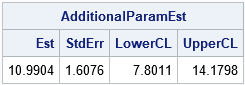The estimates and other statistics are identical to those produced by the ESTIMATE statement in PROC NLMIXED. For a general discussion of changing variables in probability and statistics, see these Penn State course notes. For a discussion about two different parameterizations of the lognormal distribution, see the blog post "Geometry, sensitivity, and parameters of the lognormal distribution."

In case you are wondering, you obtain exactly the same parameter estimates and standard errors if you fit the rate parameter directly. That is, the following call to PROC NLMIXED produces the same parameter estimates for c.

```/*Use PROC NLMIXED to fit gamma model using rate parameter */ title "Fit Gamma Model, RATE Parameter"; proc nlmixed data=Gamma; parms a 1 rate 1; * initial value for parameter; bounds 0 < a rate; model x ~ gamma(a, 1/rate); ods select ParameterEstimates; run;```

For this example, fitting the scale parameter is neither more nor less difficult than fitting the rate parameter. If I were faced with a distribution that has two different parameterizations, one simple and one more complicated, I would try to fit the simple parameters to data and use this technique to estimate the more complicated parameters.

The post Parameter estimates for different parameterizations appeared first on The DO Loop.There are several ways to use SAS to get the unique values for a data variable. In Base SAS, you can use the TABLES statement in PROC FREQ to generate a table of unique values (and the counts). You can also use the DISTINCT function in PROC SQL to get the same information. In the SAS/IML language, you can use the UNIQUE function to find the unique values of a vector. In all these cases, the values are returned in sorted (alphanumeric) order. For example, here are three ways to obtain the unique values of the TYPE variable in the Sashelp.Cars data set. Only the result from PROC IML is shown:

```/* Three ways to get unique values of a variable in sorted order */ proc freq data=sashelp.cars; tables Type / missing; /* MISSING option treats mising values as a valid category */ run;   proc sql; select distinct(Type) as uType from sashelp.cars; quit;   proc iml; use sashelp.cars; read all var "Type"; close;   uType = unique(Type); print uType;```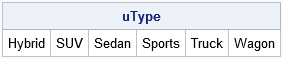Unique values in data order

The FREQ procedure has a nice option that I use FREQently: you can use the ORDER=DATA option to obtain the unique categories in the order in which they appear in a data set. Over the years, I've used the ORDER=DATA option for many purposes, including a recent post that shows how to create a "Top 10" chart. Another useful option is ORDER=FREQ, which orders the categories in descending order by frequency.

Recently a colleague asked how to obtain the unique values of a SAS/IML vector in the order in which they appear in the vector. I thought it might also be useful to support an option to return the unique values in (descending) order of frequency, so I wrote the following function. The first argument to the UniqueOrder function is the data vector. The second (optional) argument is a string that determines the order of the unique values. If the second argument has the value "DATA", then the unique values are returned in data order. If the second argument has the value "FREQ", then the unique values are returned in descending order by frequency. The function is shown below. I test the function on a character vector (TYPE) and a numeric vector (CYLINDERS) that contains some missing values.

```proc iml; /* Return the unique values in a row vector. The second parameter determines the sort order of the result. "INTERNAL": Order by alphanumeric values (default) "DATA" : Order in which elements appear in vector "FREQ" : Order by frequency */ start UniqueOrder(x, order="INTERNAL"); if upcase(order)="DATA" then do; u = unique(x); /* unique values (sorted) */ idx = j(ncol(u),1,0); /* vector to store indices in data order */ do i = 1 to ncol(u); idx[i] = loc(x=u[i]); /* 1st location of i_th unique value */ end; call sort(idx); /* sort the indices ==> data order */ return ( T(x[idx]) ); /* put the values in data order */ end; else if upcase(order)="FREQ" then do; call tabulate(u, freq, x, "missing"); /* compute freqs; missing is valid level */ call sortndx(idx, freq`) descend=1; /* order descending by freq */ return ( T(u[idx]) ); end; else return unique(x); finish;   /* test the function by calling it on a character and numeric vectors */ use sashelp.cars; read all var "Type"; read all var "Cylinders"; close;   uData = UniqueOrder(Type, "data"); /* unique values in the order they appear in data */ uFreq = UniqueOrder(Type, "freq"); /* unique values in descending frequency order */ print uData, uFreq;   uData = UniqueOrder(Cylinders, "data"); uFreq = UniqueOrder(Cylinders, "freq"); print uData, uFreq;```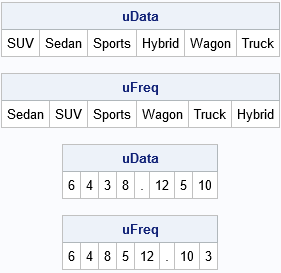The results of the function are similar to the results of PROC FREQ when you use the ORDER= option. There is a small difference when the data contain missing values. PROC FREQ always lists the missing value first, regardless of where the missing values appear in the data or how many missing values there are. In contrast, the SAS/IML function handles missing and nonmissing values equivalently.

In summary, whether you are implementing an analysis in Base SAS or SAS/IML, this article shows how to obtain the unique values of data in sorted order, in order of frequency, or in the order that the values appear in the data.

The post Get the unique values of a variable in data order appeared first on The DO Loop.This article shows how to use SAS to fit a growth curve to data. Growth curves model the evolution of a quantity over time. Examples include population growth, the height of a child, and the growth of a tumor cell. This article focuses on using PROC NLIN to estimate the parameters in a nonlinear least squares model. PROC NLIN is my first choice for fitting nonlinear parametric models to data. Other ways to model growth curves include using splines, mixed models (PROC MIXED or NLMIXED), and nonparametric methods such as loess.

The SAS DATA step specifies the mean height (in centimeters) of 58 sunflowers at 7, 14, ..., 84 days after planting. The American naturalist H. S. Reed studied these sunflowers in 1919 and used the mean height values to formulate a model for growth. Unfortunately, I do not have access to the original data for the 58 plants, but using the mean values will demonstrate the main ideas of fitting a growth model to data.

```/* Mean heights of 58 sunflowers: Reed, H. S. and Holland, R. H. (1919), "Growth of sunflower seeds" Proceedings of the National Academy of Sciences, volume 5, p. 140. http://www.pnas.org/content/pnas/5/4/135.full.pdf */ data Sunflower; input Time Height; label Time = "Time (days)" Height="Sunflower Height (cm)"; datalines; 7 17.93 14 36.36 21 67.76 28 98.1 35 131 42 169.5 49 205.5 56 228.3 63 247.1 70 250.5 77 253.8 84 254.5 ;```

Fit a logistic growth model to data

A simple mathematical model for population growth that is constrained by resources is the logistic growth model, which is also known as the Verhulst growth model. (This should not be confused with logistic regression, which predicts the probability of a binary event.) The Verhulst equation can be parameterized in several ways, but a popular parameterization is
Y(t) = K / (1 + exp(-r*(t – b)))
where

1. K is the theoretical upper limit for the quantity Y. It is called the carrying capacity in population dynamics.
2. r is the rate of maximum growth.
3. b is a time offset. The time t = b is the time at which the quantity is half of its maximum value.

The model has three parameters, K, r, and b. When you use PROC NLIN in SAS to fit a model, you need to specify the parametric form of the model and provide an initial guess for the parameters, as shown below:

```  proc nlin data=Sunflower list noitprint; parms K 250 r 1 b 40; /* initial guess */ model Height = K / (1 + exp(-r*(Time - b))); /* model to fit; Height and Time are variables in data */ output out=ModelOut predicted=Pred lclm=Lower95 uclm=Upper95; estimate 'Dt' log(81) / r; /* optional: estimate function of parameters */ run;   title "Logistic Growth Curve Model of a Sunflower"; proc sgplot data=ModelOut noautolegend; band x=Time lower=Lower95 upper=Upper95; /* confidence band for mean */ scatter x=Time y=Height; /* raw observations */ series x=Time y=Pred; /* fitted model curve */ inset ('K' = '261' 'r' = '0.088' 'b' = '34.3') / border opaque; /* parameter estimates */ xaxis grid; yaxis grid; run;```

The output from PROC NLIN includes an ANOVA table (not shown), a parameter estimates table (shown below), and an estimate for the correlation of the parameters. The parameter estimates include estimates, standard errors, and 95% confidence intervals for the parameters. The OUTPUT statement creates a SAS data set that contains the original data, the predicted values from the model, and a confidence interval for the predicted mean. The output data is used to create a "fit plot" that shows the model and the original data.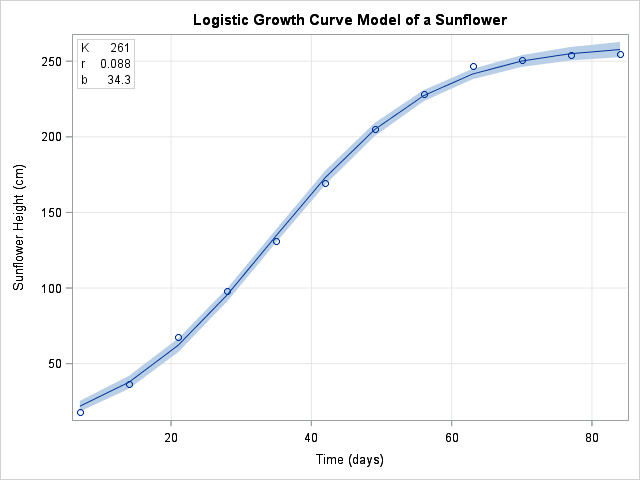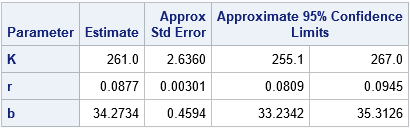Articles about the Verhulst model often mention that the "maximum growth rate" parameter, r, is sometimes replaced by a parameter that specifies the time required for the population to grow from 10% to 90% of the carrying capacity, K. This time period is called the "characteristic duration" and denoted as Δt. You can show that Δt = log(81)r. The ESTIMATE statement in PROC NLIN produces a table (shown below) that estimates the characteristic duration.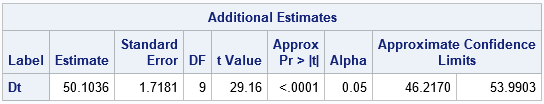The value 50.1 tells you that, on average, it takes about 50 days for a sunflower to grow from 10 percent of its maximum height to 90 percent of its maximum height. By looking at the graph, you can see that most growth occurs during the 50 days between Day 10 and Day 60.

This use of the ESTIMATE statement can be very useful. Some models have more than one popular parameterization. You can often fit the model for one parameterization and use the ESTIMATE statement to estimate the parameters for a different parameterization.

The post Fit a growth curve in SAS appeared first on The DO Loop.Programmers on a SAS discussion forum recently asked about the chi-square test for proportions as implemented in PROC FREQ in SAS. One person asked the basic question, "how do I test the null hypothesis that the observed proportions are equal to a set of known proportions?" Another person said that the null hypothesis was rejected for his data, and he wanted to know which categories were "responsible for the rejection." This article answers both questions and points out a potential pitfall when you specify the proportions for a chi-square goodness-of-fit test in PROC FREQ.

The basic idea: The proportion of party affiliations for a group of voters

To make these questions concrete, let's look at some example data. According to a 2016 Pew research study, the party affiliation of registered voters in the US in 2016 was as follows: 33% of voters registered as Democrats, 29% registered as Republicans, 34% were Independents, and 4% registered as some other party. If you have a sample of registered voters, you might want to ask whether the observed proportion of affiliations matches the national averages. The following SAS data step defines the observed frequencies for a hypothetical sample of 300 voters:

```data Politics; length Party \$5; input Party \$ Count; datalines; Dem 125 Repub 79 Indep 86 Other 10 ;```

You can use the TESTP= option on the TABLES statement in PROC FREQ to compare the observed proportions with the national averages for US voters. You might assume that the following statements perform the test, but there is a potential pitfall. The following statements contain a subtle error:

```proc freq data=Politics; /* National Pct: D=33%, R=29%, I=34%, Other=04% */ tables Party / TestP=(0.33 0.29 0.34 0.04) nocum; /* WARNING: Contains an error! */ weight Count; run;```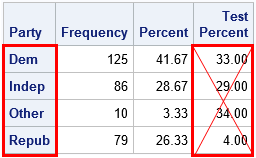If you look carefully at the OneWayFreqs table that is produced, you will see that the test proportions that appear in the fourth column are not the proportions that we intended to specify! The problem is that order of the categories in the table is alphabetical whereas the proportions in the LISTP= option correspond to the order that the categories appear in the data. In an effort to prevent this mistake, the documentation for the TESTP= option warns you to "order the values to match the order in which the corresponding variable levels appear in the one-way frequency table." The order of categories is important in many SAS procedures, so always think about the order! (The ESTIMATE and CONTRAST statements in linear regression procedures are other statements where order is important.)

Specify the test proportions correctly

To specify the correct order, you have two options: (1) list the proportions for the TESTP= option according to the alphabetical order of the categories, or (2) use the ORDER=DATA option on the PROC FREQ statement to tell the procedure to use the order of the categories as they appear in the data. The following statement uses the ORDER=DATA option to specify the proportions:

```proc freq data=Politics ORDER=DATA; /* list proportions in DATA order */ /* D=33%, R=29%, I=34%, Other=04% */ tables Party / TestP=(0.33 0.29 0.34 0.04); /* cellchi2 not available for one-way tables */ weight Count; ods output OneWayFreqs=FreqOut; output out=FreqStats N ChiSq; run;```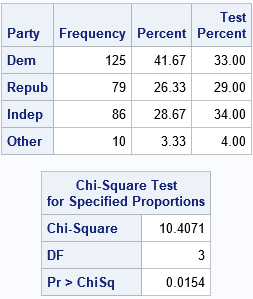The analysis is now correctly specified. The chi-square table indicates that the observed proportions are significantly different from the national averages at the α = 0.05 significance level.

Which categories are "responsible" for rejecting the null hypothesis?

A SAS programmer posted a similar analysis on a discussion and asked whether it was possible to determine which categories were the most different from the specified proportions. The analysis shows that the chi-square test rejects the null hypothesis, but does not indicate whether only one category is different than expected or whether many categories are different.

Interestingly, PROC FREQ supports such an option for two-way tables when the null hypothesis is the independence of the two variables. Recall that the chi-square statistic is a sum of squares, where each cell in the table contributes one squared value to the sum. The CELLCHI2 option on the TABLES statement "displays each table cell’s contribution to the Pearson chi-square statistic.... The cell chi-square is computed as
(frequencyexpected)2 / expected
where frequency is the table cell frequency (count) and expected is the expected cell frequency" under the null hypothesis.

Although the option is not supported for one-way tables, it is straightforward to use the DATA step to compute each cell's contribution. The previous call to PROC FREQ used the ODS OUTPUT statement to write the OneWayFreqs table to a SAS data set. It also wrote a data set that contains the sample size and the chi-square statistic. You can use these statistics as follows:

```/* create macro variables for sample size and chi-square statistic */ data _NULL_; set FreqStats; call symputx("NumObs", N); call symputx("TotalChiSq", _PCHI_); run;   /* compute the proportion of chi-square statistic that is contributed by each cell in the one-way table */ data Chi2; set FreqOut; ExpectedFreq = &NumObs * TestPercent / 100; Deviation = Frequency - ExpectedFreq; ChiSqContrib = Deviation**2 / ExpectedFreq; /* (O - E)^2 / E */ ChiSqPropor = ChiSqContrib / &TotalChiSq; /* proportion of chi-square contributed by this cell */ format ChiSqPropor 5.3; run;   proc print data=Chi2; var Party Frequency TestPercent ExpectedFreq Deviation ChiSqContrib ChiSqPropor; run;```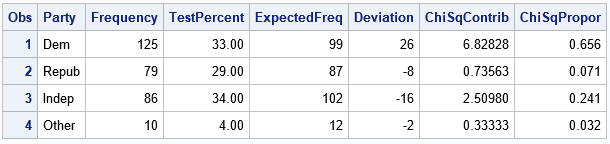The table shows the numbers used to compute the chi-square statistic. For each category of the PARTY variable, the table shows the expected frequencies, the deviations from the expected frequencies, and the chi-square term for each category. The last column is the proportion of the total chi-square statistic for each category. You can see that the 'Dem' category contributes the greatest proportion. The interpretation is that the observed count of the 'Dem' group is much greater than expected and this is the primary reason why the null hypothesis is rejected.

You can also create a bar chart that shows the contributions to the chi-square statistic. You can create the "chi-square contribution plot" by using the following statements:

```title "Proportion of Chi-Square Statistic for Each Category"; proc sgplot data=Chi2; vbar Party / response=ChiSqPropor datalabel=ChiSqPropor; xaxis discreteorder=data; yaxis label="Proportion of Chi-Square Statistic" grid; run;```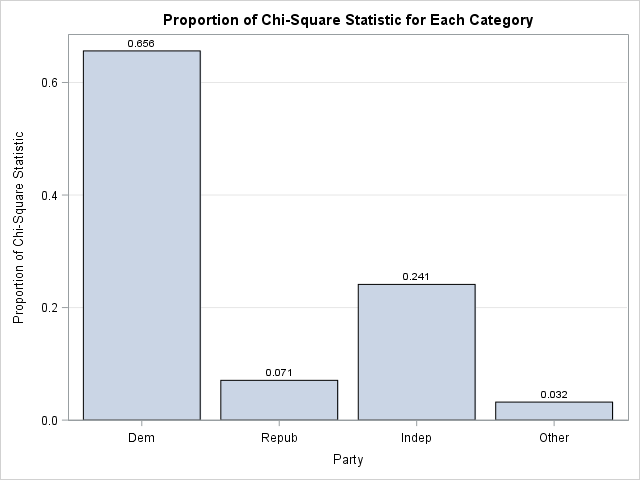The bar chart makes it clear that the frequency of the 'Dem' group is the primary factor in the size of the chi-square statistic. The "chi-square contribution plot" is a visual companion to the Deviation Plot, which is produced automatically by PROC FREQ when you specify the PLOTS=DEVIATIONPLOT option. The Deviation Plot shows whether the counts for each category are more than expected or less than expected. When you combine the two plots, you can make pronouncements like Goldilocks:

• The 'Dem' group contributes the most to the chi-square statistic because the observed counts are "too big."
• The 'Indep' group contributes a moderate amount because the counts are "too small."
• The remaining groups do not contribute much because their counts are "just right."

Summary

In summary, this article addresses three topics related to testing the proportions of counts in a one-way frequency table. You can use the TESTP= option to specify the proportions for the null hypothesis. Be sure that you specify the proportions in the same order that they appear in the OneWayFreqs table. (The ORDER=DATA option is sometimes useful for this.) If the data proportions do not fit the null hypothesis, you might want to know why. One way to answer this question is to compute the contributions of each category to the total chi-square computation. This article shows how to display that information in a table or in a bar chart.

The post Chi-square tests for proportions in one-way tables appeared first on The DO Loop.Have you ever tried to type a movie title by using a TV remote control? Both Netflix and Amazon Video provide an interface (a virtual keyboard) that enables you to use the four arrow keys of a standard remote control to type letters. The letters are arranged in a regular grid and to navigate from one letter to another can require you to press the arrow keys many times. Fortunately, the software displays partial matches as you choose each letter, so you rarely need to type the entire title. Nevertheless, I was curious: Which interface requires the fewest number of key presses (on average) to type a movie title by using only the arrow keys?

The layout of the navigation screens

The following images show the layout of the navigation screen for Netflix and for Amazon Video.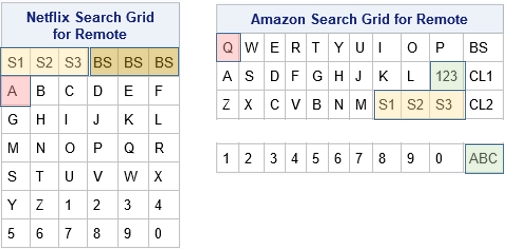The Netflix grid has 26 letters and 10 numbers arranged in a 7 x 6 grid. The letters are arranged in alphabetical order. The first row contains large keys for the Space character (shown in a light yellow color) and the Backspace key (dark yellow). Each of those keys occupies three columns of the grid, which means that you can get to the Space key by pressing Up Arrow from the A, B, or C key. When you first arrive at the Netflix navigation screen, the cursor is positioned on the A key (shown in pink).

The letters in the Amazon Video grid are arranged in a 3 x 11 grid according to the standard QWERTY keyboard layout. When you first arrive at the navigation screen, the cursor is positioned on the Q key. The Space character can be typed by using a large key in the last row (shown in a light yellow color) that spans columns 8, 9, and 10. The Space character can be accessed from the M key (press Right Arrow) or from the K, L, or '123' keys on the second row. The '123' key (shown in green) navigates to a second keyboard that contains numbers and punctuation. The numbers are arranged in a 1 x 11 grid. When you arrive at that keyboard, the cursor is on the 'ABC' key, which takes you back to the keyboard that contains letters. (Note: The real navigation screen places the '123' key under the 0 key. However, the configuration in the image is equivalent because in each case you must press one arrow key to get to the 0 (zero) key.) For simplicity, this article ignores punctuation in movie titles.

Which navigation interface is more efficient?

I recently wrote a mathematical discussion about navigating through grids by moving only Up, Down, Left, and Right. The article shows that nearly square grids are more efficient than short and wide grids, assuming that the letters that you type are chosen at random. A 7 x 6 grid requires an average of 4.23 key presses per character whereas a 4 x 11 grid requires an average of 4.89 key presses per character. Although the difference might not seem very big, the average length of a movie title is about 15 characters (including spaces). For a 15-character title, the mathematics suggests that using the Netflix interface requires about 10 fewer key presses (on average) than the Amazon Video interface.

If you wonder why I did not include the Hulu interface in this comparison, it is because the Hulu "keyboard" is a 1 x 28 grid that contains all letters and the space and backspace keys. Theory predicts an average of 9.32 key presses per character, which is almost twice as many key presses as for the Netflix interface.

Comparing two interfaces for selecting movie titles

You might wonder how well this theoretical model matches reality. Movie titles are not a jumble of random letters! How do the Netflix and Amazon Video interfaces compare when they are used to type actual movie titles?

To test this question, I downloaded the titles of 1,000 highly-rated movies. I wrote a SAS program that calculates the number of the arrow keys that are needed to type each movie title for each interface. This section summarizes the results.

The expression "press the arrow key," is a bit long, so I will abbreviate it as "keypress" (one word). The "number of times that you need to press the arrow keys to specify a movie title" is similarly condensed to "the number of keypresses."

For these 1,000 movie titles, the Netflix interface requires an average of 50.9 keypresses per title or 3.32 keypresses per character. the Amazon Video interface requires an average of 61.4 keypresses per title or 4.01 keypresses per character. Thus, on average, the Netflix interface requires 10.56 fewer keypresses per title, which closely agrees with the mathematical prediction that consider only the shape of the keyboard interface. A paired t test indicates that the difference between the means is statistically significant. The difference between medians is similar: 45 for the Netflix interface and 56 for the Amazon interface.

The following comparative histogram (click to enlarge) shows the distribution of the number of keypresses for each of the 1,000 movie titles for each interface. The upper histogram shows that most titles require between 30 and 80 keypresses in the Amazon interface, with a few requiring more than 140 keypresses. In contrast, the lower histogram indicates that most titles require between 20 and 60 keypresses in the Netflix interface; relatively fewer titles require more than 140 keypresses.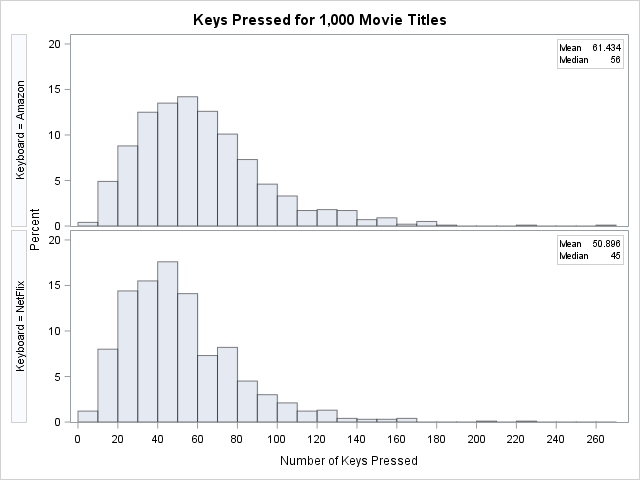You can also use a scatter plot to compare the number of keypresses that are required for each interface. Each marker in the following scatter plot shows the number of keypresses for a title in the Amazon interface (horizontal axis) and the Netflix interface (vertical axis). Markers that are below and to the right of the diagonal (45-degree) line are titles for which the Netflix interface requires fewer keypresses. Markers that are above and to the left of the diagonal line are titles for which the Amazon interface is more efficient. You can see that most markers are below the diagonal line. In fact, 804 titles require fewer keypresses in the Netflix interface, only 177 favor the Amazon interface, and 19 require the same number of keypresses in both interfaces. Clearly, the Netflix layout of the virtual keyboard is more efficient for specifying movie titles.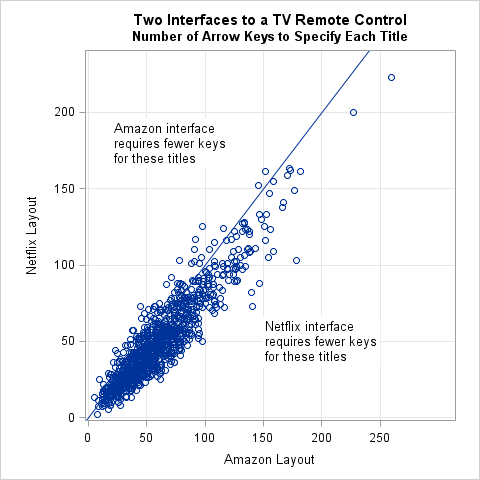Movie titles that require the most and the fewest key presses

The scatter plot and histograms reveal that there are a few movies whose titles require many keypresses. Here is a list of the 10 titles that require the most keypresses when using the Amazon interface: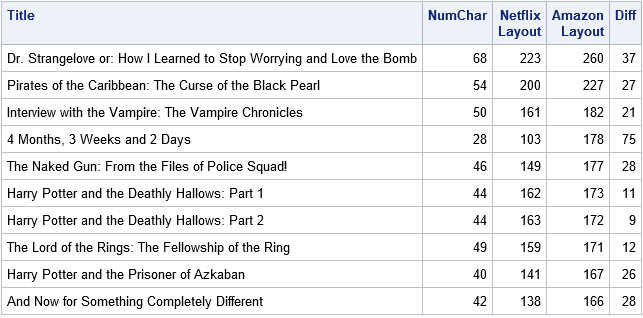Most of the titles are long. However, one (4 Months, 3 Weeks and 2 Days) is not overly long but instead requires shifting back and forth between the two keyboards in the Amazon interface. That results in a large number of keypresses in the Amazon interface (178) and a large difference between the keypresses required by each interface. In fact, the absolute difference for that title (75) is the largest difference among the 1,000 titles.

You can also look at the movie titles that require few keypresses. The following table shows titles that require fewer than 10 keypresses in either interface. The titles that require the fewest keypresses in the Netflix interface are M, Moon, PK, and Up. The titles that require the fewest keypresses in the Amazon interface are Saw, M, Creed, and Up. It is interesting that Saw, which has three letters, requires fewer keypresses than M, which has one letter. That is because the S, A, and W letters are all located in the upper left of the QWERTY keyboard whereas the letter M is in the lower left corner of the keyboard. (Recall that the cursor begins on the Q letter in the upper left corner.).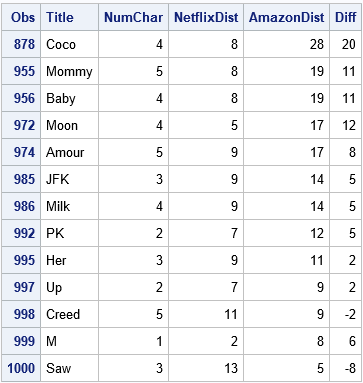Summary

In summary, both Netflix and Amazon Video provide an interface that enables customers to select movie titles by using the four arrow keys on a TV remote control. The Netflix interface is a 7 x 6 grid of letters; the Amazon interface is a 3 x 11 QWERTY keyboard and a separate keyboard for numbers. In practice, both interfaces display partial matches and you only need to type a few characters. However, it is interesting to statistically compare the interfaces in terms of efficiency. For a set of 1,000 movie titles, the Netflix interface requires, on average, 10.6 fewer keypresses than the Amazon interface to completely type the titles. This article also lists the movie titles that require the most and the fewest number of key presses.

If you would like to duplicate or extend this analysis, you can download the SAS program that contains the data.

The post Two interfaces for typing text by using a TV remote control appeared first on The DO Loop.A frequent topic on SAS discussion forums is how to check the assumptions of an ordinary least squares linear regression model. Some posts indicate misconceptions about the assumptions of linear regression. In particular, I see incorrect statements such as the following:

• Help! A histogram of my variables shows that they are not normal! I need to apply a normalizing transformation before I can run a regression....
• Before I run a linear regression, I need to test that my response variable is normal....

Let me be perfectly clear: The variables in a least squares regression model do not have to be normally distributed. I'm not sure where this misconception came from, but perhaps people are (mis)remembering an assumption about the errors in an ordinary least squares (OLS) regression model. If the errors are normally distributed, you can prove theorems about inferential statistics such as confidence intervals and hypothesis tests for the regression coefficients. However, the normality-of-errors assumption is not required for the validity of the parameter estimates in OLS. For the details, the Wikipedia article on ordinary least squares regression lists four required assumptions; the normality of errors is listed as an optional fifth assumption.

In practice, analysts often "check the assumptions" by running the regression and then examining diagnostic plots and statistics. Diagnostic plots help you to determine whether the data reveal any deviations from the assumptions for linear regression. Consequently, this article provides a "getting started" example that demonstrates the following:

1. The variables in a linear regression do not need to be normal for the regression to be valid.
2. You can use the diagnostic plots that are produced automatically by PROC REG in SAS to check whether the data seem to satisfy some of the linear regression assumptions.

By the way, don't feel too bad if you misremember some of the assumptions of linear regression. Williams, Grajales, and Kurkiewicz (2013) point out that even professional statisticians sometimes get confused.

An example of nonnormal data in regression

Consider this thought experiment: Take any explanatory variable, X, and define Y = X. A linear regression model perfectly fits the data with zero error. The fit does not depend on the distribution of X or Y, which demonstrates that normality is not a requirement for linear regression.

For a numerical example, you can simulate data such that the explanatory variable is binary or is clustered close to two values. The following data shows an X variable that has 20 values near X=5 and 20 values near X=10. The response variable, Y, is approximately five times each X value. (This example is modified from an example in Williams, Grajales, and Kurkiewicz, 2013.) Neither variable is normally distributed, as shown by the output from PROC UNIVARIATE:

```/* For n=1..20, X ~ N(5, 1). For n=21..40, X ~ N(10, 1). Y = 5*X + e, where e ~ N(0,1) */ data Have; input X Y @@; datalines; 3.60 16.85 4.30 21.30 4.45 23.30 4.50 21.50 4.65 23.20 4.90 25.30 4.95 24.95 5.00 25.45 5.05 25.80 5.05 26.05 5.10 25.00 5.15 26.45 5.20 26.10 5.40 26.85 5.45 27.90 5.70 28.70 5.70 29.35 5.90 28.05 5.90 30.50 6.60 33.05 8.30 42.50 9.00 45.50 9.35 46.45 9.50 48.40 9.70 48.30 9.90 49.80 10.00 48.60 10.05 50.25 10.10 50.65 10.30 51.20 10.35 49.80 10.50 53.30 10.55 52.15 10.85 56.10 11.05 55.15 11.35 55.95 11.35 57.90 11.40 57.25 11.60 57.95 11.75 61.15 ;   proc univariate data=Have; var x y; histogram x y / normal; run;```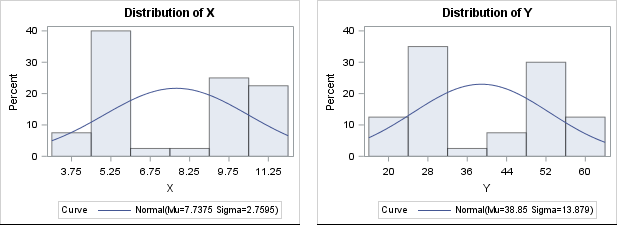There is no need to "normalize" these data prior to performing an OLS regression, although it is always a good idea to create a scatter plot to check whether the variables appear to be linearly related. When you regress Y onto X, you can assess the fit by using the many diagnostic plots and statistics that are available in your statistical software. In SAS, PROC REG automatically produces a diagnostic panel of graphs and a table of fit statistics (such as R-squared):

```/* by default, PROC REG creates a FitPlot, ResidualPlot, and a Diagnostics panel */ ods graphics on; proc reg data=Have; model Y = X; quit;```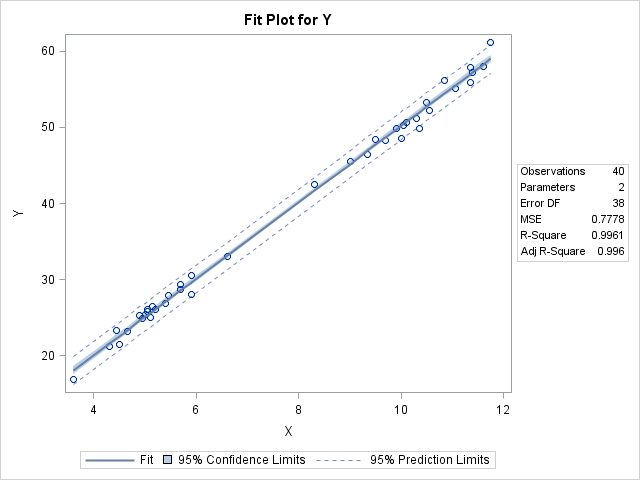The R-squared value for the model is 0.9961, which is almost a perfect fit, as seen in the fit plot of Y versus X.

Using diagnostic plots to check the assumptions of linear regression

You can use the graphs in the diagnostics panel to investigate whether the data appears to satisfy the assumptions of least squares linear regression. The panel is shown below (click to enlarge).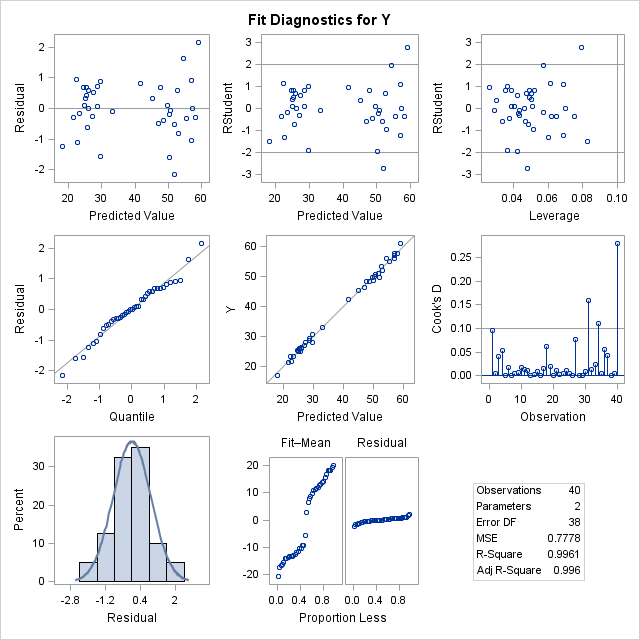The first column in the panel shows graphs of the residuals for the model. For these data and for this model, the graphs show the following:

• The top-left graph shows a plot of the residuals versus the predicted values. You can use this graph to check several assumptions: whether the model is specified correctly, whether the residual values appear to be independent, and whether the errors have constant variance (homoscedastic). The graph for this model does not show any misspecification, autocorrelation, or heteroscedasticity.
• The middle-left and bottom-left graphs indicate whether the residuals are normally distributed. The middle plot is a normal quantile-quantile plot. The bottom plot is a histogram of the residuals overlaid with a normal curve. Both these graphs indicate that the residuals are normally distributed. This is evidence that you can trust the p-values for significance and the confidence intervals for the parameters.